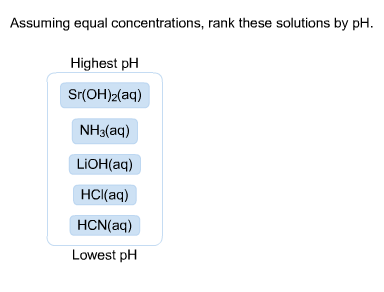# (SOLVED) Assuming equal concentrations, rank these solutions by pH.

Discipline: Chemistry

Paper Format: APA

Pages: 1 Words: 275

Question

Assuming equal concentrations, rank these solutions by pH Highest pH Sr(OH)2(aq) NH3(aq) LiOH(aq) HCl(aq) HCN(aq) Lowest pHHighest PH

Sr(OH)2(aq)

LiOH(aq)

NH3(aq)

HCN(aq)

HCl(aq)

Lowest PH

PH IS MORE FOR MORE BASIC SOLUTIONS AND PH IS LESS FOR MORE ACIDIC SOLUTIONS